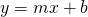# Appliance Repair

Here is a set of points showing the relationship between how long an appliance repair technician works in a home and how much their visit costs.

(a) How much does it cost to have the technician arrive at your house?

(b) How much does the fee increase for each hour?

(c) Which variable – hours or cost –  is the independent variable?

(d) What is the rate of change?

(e) How much does 4 hours cost?

(f) How much does 10 hours cost?

(g) How much does ‘x’ hours cost?

(h) What equation can you write in the formthat goes through these points?

Try your equation out in the applet above.

Linear Relations 11 AW Unit 2